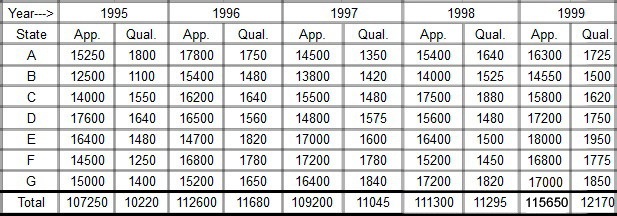## RBI Assistant Quant Test 8

Instructions

Study the following table carefully and answer the questions given below it.
Number of candidates appeared and qualified in a competitive examination from different states over the years.Q 1

What is the percentage of candidates qualified in 1998 and 1999 together from all the states w.r.t. the candidates appeared from all the states in these two years (the value upto two decimal points)?

Q 2

For which of the following states the percentage of candidate qualified w.r.t. those appeared is the lowest in the year 1995 ?

Q 3

What approximately is the percentage of candidates qualified from states C and D together w.r.t. the candidates appeared from these two states in 1997 ?

Q 4

What approximately is the average number of candidates qualified in 1998 from the given states ?

Q 5

What is the average number of candidates appeared from state B in the given years ?